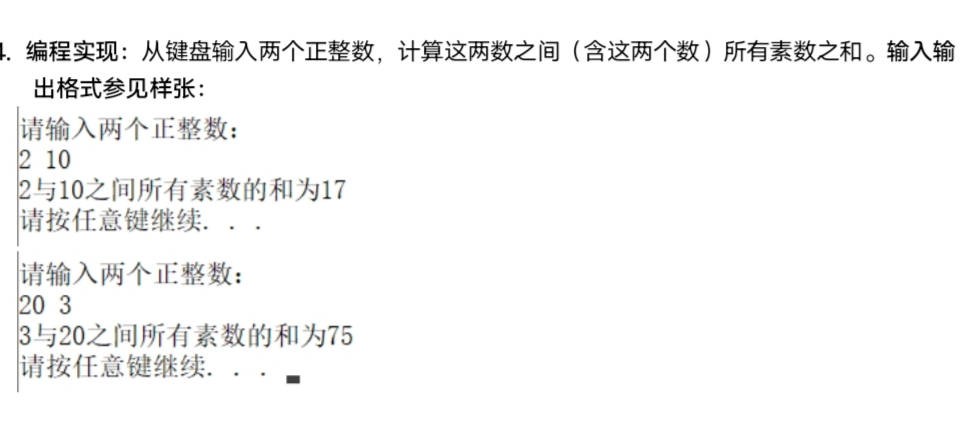2021-10-19 22:59

# 这个题目怎么做啊？c++的题目😭• 好问题 提建议
• 收藏

#### 1条回答默认 最新

•yumueat 2021-10-20 13:35
已采纳
``````
#include <iostream>
#include <math.h>
using namespace std;
int ans(int a)//判断是否是素数的函数
{
for(int i=2;i<=sqrt(a);i++)//判断到sqrt（a）就够了
{
if (a%i==0)
{
return 0;//不是素数就返回0，这样直接相加也没有影响
}
}
return a;//是素数就返回本身
}
int main()
{

int a,b,i,s=0,temp;
cin>>a>>b;
if (a>b)
{
temp=a;
a=b;
b=temp;
}
for (i=a;i<=b;i++)
{
s+= ans(i);//对素数求和
}
printf("%d和%d之间所有素数的和为%d",a,b,s);
return 0;
}
``````
已采纳该答案
评论
解决 1 无用
打赏 举报